PSA2QS3 - Classwork---Geometry, M1, Lesson 6 (G.CO.9)
Part A)

Determine the measure of the missing angle in the diagram.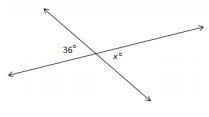Modified from EngageNY ©Great Minds Disclaimer

Type your answer below as a number (example: 5, 3.1, 4 1/2, or 3/2):
Part B)

What facts about angles did you use?

Part A)

Determine the measure of the missing angle in each diagram.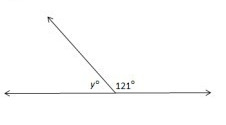Modified from EngageNY ©Great Minds Disclaimer

Type your answer below as a number (example: 5, 3.1, 4 1/2, or 3/2):
Part B)

What facts about angles did you use?

Part A)

Determine the measure of the missing angle in each diagram.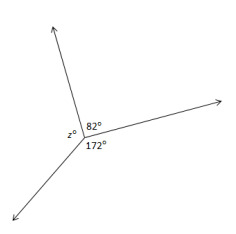Modified from EngageNY ©Great Minds Disclaimer

Type your answer below as a number (example: 5, 3.1, 4 1/2, or 3/2):
Part B)

What facts about angles did you use?

Part A)

Two angles, with a common side,are ___________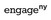Modified from EngageNY ©Great Minds Disclaimer

Part B)

Two angles, with a common side,are adjacent angles if C belongs to the interior of. The sum of angles on a straight line is 180°, and two such angles are called a linear pair. Two angles are called supplementary if the sum of their measures is ______________°

Type your answer below as a number (example: 5, 3.1, 4 1/2, or 3/2):
Part C)

Two angles, with a common side,are adjacent angles if C belongs to the interior of. The sum of angles on a straight line is 180°, and two such angles are called a linear pair. Two angles are called supplementary if the sum of their measures is 180°; two angles are called complementary if the sum of their measures is

_______________°

Describing angles as supplementary or complementary refers only to the measures of their angles. The positions of the angles or whether the pair of angles is adjacent to each other is not part of the definition

Type your answer below as a number (example: 5, 3.1, 4 1/2, or 3/2):

In the figure, Line segment AD is drawn. Find.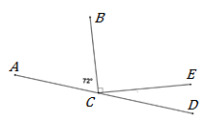Modified from EngageNY ©Great Minds Disclaimer

Type your answer below as a number (example: 5, 3.1, 4 1/2, or 3/2):

The total measure of adjacent angles around a point is ___________ °

Modified from EngageNY ©Great Minds Disclaimer

Type your answer below as a number (example: 5, 3.1, 4 1/2, or 3/2):

Find the measure of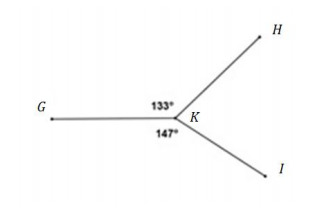Modified from EngageNY ©Great Minds Disclaimer

Type your answer below as a number (example: 5, 3.1, 4 1/2, or 3/2):

Vertical angles have _________ measure.  Two angles are vertical if their sides form opposite rays.

Modified from EngageNY ©Great Minds Disclaimer

Find.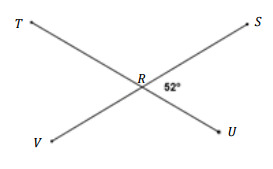Modified from EngageNY ©Great Minds Disclaimer

Type your answer below as a number (example: 5, 3.1, 4 1/2, or 3/2):
Part A)

Find the measure of the labeled angle.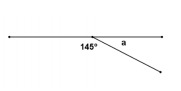Angle a = ____.

Modified from EngageNY ©Great Minds Disclaimer

Type your answer below as a number (example: 5, 3.1, 4 1/2, or 3/2):
Part B)

Give a reason for your solution.

Part A)

Find the measure of each labeled angle.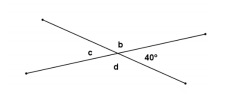Angle b = ____.

Modified from EngageNY ©Great Minds Disclaimer

Type your answer below as a number (example: 5, 3.1, 4 1/2, or 3/2):
Part B)

Give a reason for your solution.

Part C)

Angle b = ____.

Type your answer below as a number (example: 5, 3.1, 4 1/2, or 3/2):
Part D)

Give a reason for your solution.

Part E)

Angle d = ____.

Type your answer below as a number (example: 5, 3.1, 4 1/2, or 3/2):
Part F)

Give a reason for your solution.

Part G)
Type your answer below as a number (example: 5, 3.1, 4 1/2, or 3/2):
Part A)

Find the measure of the labeled angle.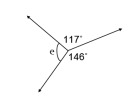Angle e = ____.

Modified from EngageNY ©Great Minds Disclaimer

Part B)

Give a reason for your solution.

Part A)

In the figure below,is a straight line segment. Find the measure of the marked angle, or find the unknown number labeled by the variables in the diagram.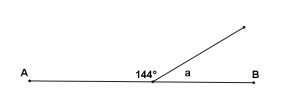=___________

Modified from EngageNY ©Great Minds Disclaimer

Type your answer below as a number (example: 5, 3.1, 4 1/2, or 3/2):
Part B)

Give reasons for your calculations. Show all the steps to your solutions.

Write your work on paper, take a picture, and upload it using the image upload icon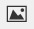.

If you do not have the ability to upload an image of your work, type "Work is on paper."

Part A)

In the figure below,is a straight line segment. Find the measure of the marked angle, or find the unknown number labeled by the variables in the diagram. Give reasons for your calculations. Show all the steps to your solution.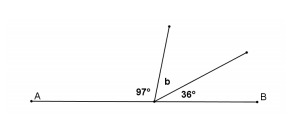=___________

Modified from EngageNY ©Great Minds Disclaimer

Type your answer below as a number (example: 5, 3.1, 4 1/2, or 3/2):
Part B)

Give reasons for your calculations. Show all the steps to your solutions.

Write your work on paper, take a picture, and upload it using the image upload icon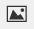.

If you do not have the ability to upload an image of your work, type "Work is on paper."

Part A)

In the figure below,is a straight line segment. Find the measure of the marked angle, or find the unknown number labeled by the variables in the diagram. Give reasons for your calculations. Show all the steps to your solution.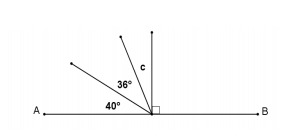=___________

Modified from EngageNY ©Great Minds Disclaimer

Type your answer below as a number (example: 5, 3.1, 4 1/2, or 3/2):
Part B)

Give reasons for your calculations. Show all the steps to your solutions.

Write your work on paper, take a picture, and upload it using the image upload icon.

If you do not have the ability to upload an image of your work, type "Work is on paper."

Part A)

In the figure below,is a straight line segment. Find the measure of the marked angle, or find the unknown number labeled by the variables in the diagram. Give reasons for your calculations. Show all the steps to your solution.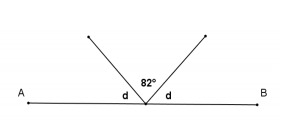=___________

Modified from EngageNY ©Great Minds Disclaimer

Type your answer below as a number (example: 5, 3.1, 4 1/2, or 3/2):
Part B)

Give reasons for your calculations. Show all the steps to your solutions.

Write your work on paper, take a picture, and upload it using the image upload icon.

If you do not have the ability to upload an image of your work, type "Work is on paper."

Part C)
Type your answer below as a number (example: 5, 3.1, 4 1/2, or 3/2):
Part A)

In the figure below,is a straight line segment. Find the measure of the marked angle, or find the unknown number labeled by the variables in the diagram. Give reasons for your calculations. Show all the steps to your solution.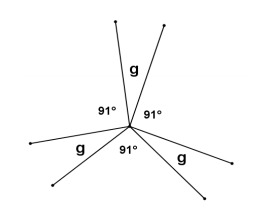=___________

Modified from EngageNY ©Great Minds Disclaimer

Type your answer below as a number (example: 5, 3.1, 4 1/2, or 3/2):
Part B)

Give reasons for your calculations. Show all the steps to your solutions.

Write your work on paper, take a picture, and upload it using the image upload icon.

If you do not have the ability to upload an image of your work, type "Work is on paper."

Part C)
Type your answer below as a number (example: 5, 3.1, 4 1/2, or 3/2):
Part A)

Find the value of x. Show all the work.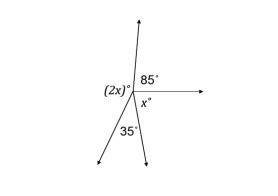x = ______

Modified from EngageNY ©Great Minds Disclaimer

Type your answer below as a number (example: 5, 3.1, 4 1/2, or 3/2):
Part B)

Write your work on paper, take a picture, and upload it using the image upload icon.

If you do not have the ability to upload an image of your work, type "Work is on paper."

Part A)

Find the values of x and y. Show all the work.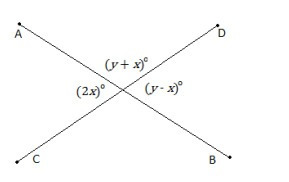x = _________Modified from EngageNY ©Great Minds Disclaimer

Type your answer below as a number (example: 5, 3.1, 4 1/2, or 3/2):
Part B)

Find the values of x and y. Show all the work.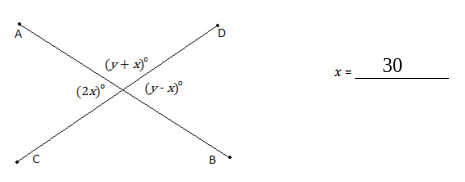y = _________

Type your answer below as a number (example: 5, 3.1, 4 1/2, or 3/2):
Part C)

Write your work on paper, take a picture, and upload it using the image upload icon.

If you do not have the ability to upload an image of your work, type "Work is on paper."

Part A)

Find the values of x and y. Show all the work.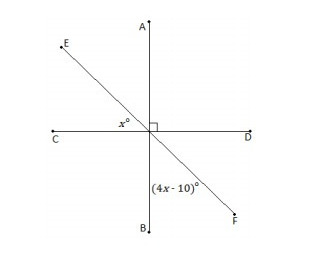x = _________Modified from EngageNY ©Great Minds Disclaimer

Type your answer below as a number (example: 5, 3.1, 4 1/2, or 3/2):
Part B)

Write your work on paper, take a picture, and upload it using the image upload icon.

If you do not have the ability to upload an image of your work, type "Work is on paper."

Part A)

Find the values of x and y. Show all the work.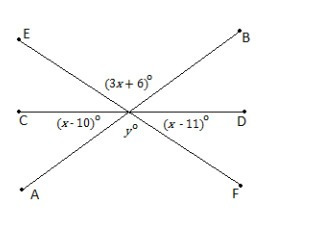x = _________Modified from EngageNY ©Great Minds Disclaimer

Type your answer below as a number (example: 5, 3.1, 4 1/2, or 3/2):
Part B)

Find the values of x and y. Show all the work.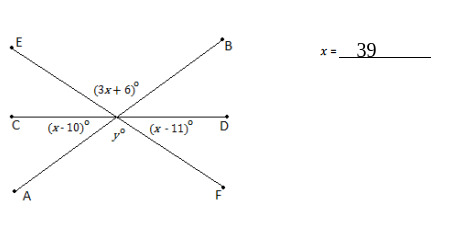y = _________

Type your answer below as a number (example: 5, 3.1, 4 1/2, or 3/2):
Part C)

Write your work on paper, take a picture, and upload it using the image upload icon.

If you do not have the ability to upload an image of your work, type "Work is on paper."

Part A)

Find the values of x and y. Show all the work.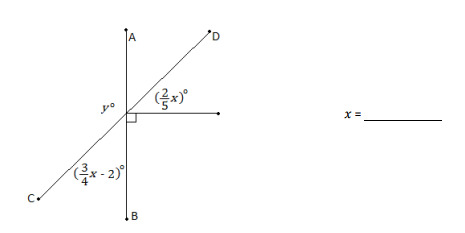x = _________Modified from EngageNY ©Great Minds Disclaimer

Type your answer below as a number (example: 5, 3.1, 4 1/2, or 3/2):
Part B)

Find the values of x and y. Show all the work.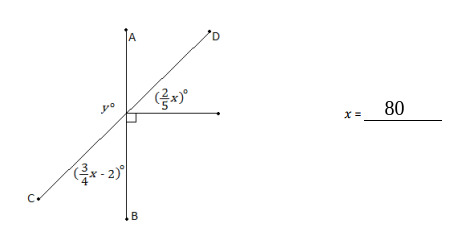y = _________

Type your answer below as a number (example: 5, 3.1, 4 1/2, or 3/2):
Part C)

Write your work on paper, take a picture, and upload it using the image upload icon.

If you do not have the ability to upload an image of your work, type "Work is on paper."

Part A)

Find the values of x and y. Show all the work.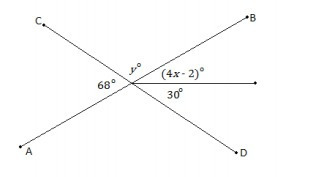x = _________Modified from EngageNY ©Great Minds Disclaimer

Type your answer below as a number (example: 5, 3.1, 4 1/2, or 3/2):
Part B)

Find the values of x and y. Show all the work.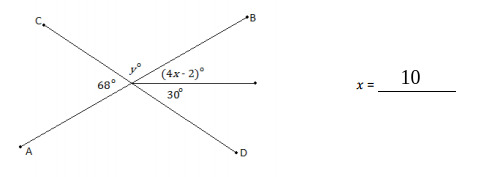y = _________

Type your answer below as a number (example: 5, 3.1, 4 1/2, or 3/2):
Part C)

Write your work on paper, take a picture, and upload it using the image upload icon.

If you do not have the ability to upload an image of your work, type "Work is on paper."

Part A)

Find the values of x and y. Show all the work.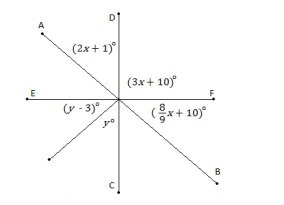x = _________Modified from EngageNY ©Great Minds Disclaimer

Type your answer below as a number (example: 5, 3.1, 4 1/2, or 3/2):
Part B)

Find the values of x and y. Show all the work.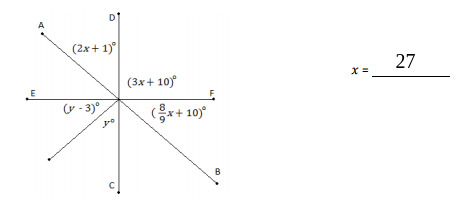y = _________Type your answer below as a number (example: 5, 3.1, 4 1/2, or 3/2):
Part C)

Write your work on paper, take a picture, and upload it using the image upload icon.

If you do not have the ability to upload an image of your work, type "Work is on paper."